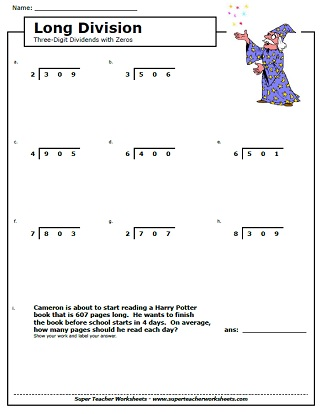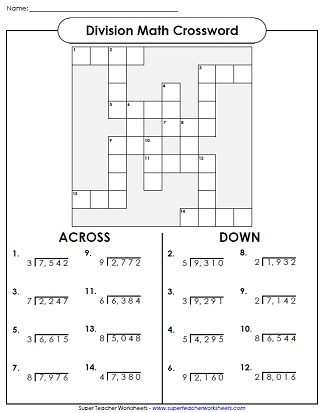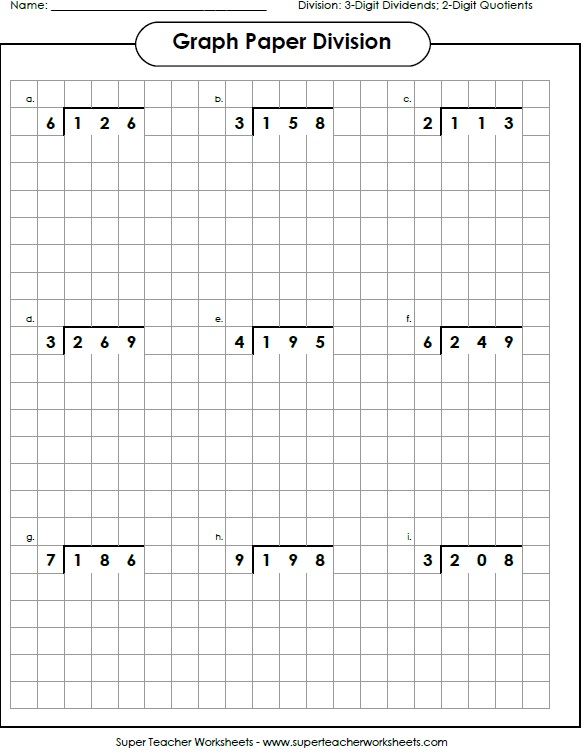# Long Division Worksheets

The printable worksheets on this page feature simple division with remainders, as well as long division problems.

If you'd like to view the Common Core Standard(s) associated with the long division worksheets below, simply click the common core icon.

1-Digit and 2-Digit Dividends
Solve these division problems; includes 1-digit and 2-digit quotients with remainders. Then color the picture of the knight, dragon, and castle.
1 and 2-digit quotients, all problems have remainders; Winter snowmen pictures on the page.
Student rewrite each division problem and solve. Each problem has a 2-digit dividend. The quotients each have 1 or 2 digits. Most problems have remainders.
2 or 3-digit dividends, 2 or 3-digit quotient, problems do not have remainders; Lion picture and word problem.
Division problems with 2-digit dividends and 2-digit quotients.
3-Digit Dividends
Find the answers to the division problems and color the quotients on the bingo board.  Can you get a bingo?  (3-digit dividends and 2-digit quotients without remainders.)
3-digit dividends, 2 or 3-digit quotient, all problems have remainders; Goldfish picture and word problem.
This worksheet has a math riddle for students to solve. What do you call a sleeping bull? To decipher the answer, students will solve division problems with 3-dig dividends and 2-dig quotients. These problems do not have remainders.
Divide the problem on each task card. Each problem has a 3-digit dividend and a 1-digit divisor. (example: 126 divided by 6) These cards can be used for classroom scavenger hunts, learning centers, document readers, or small group instruction.
What do you call a cow on your front lawn? The answer to the riddle can be solved by completing division problems. Each problem has a 3-digit dividend. All quotients have remainders.
Division problems with 3-digit dividends; with Remainders; Crab pictures.
Division problems with 3-digit dividends; some have remainders; Students need to re-write the problem in long division form.
Student rewrite each long division division problem and solve. Each problem has a 3-digit dividend.
At the top of the worksheet, students are shown a series of shapes with numbers in them. They divide numbers in similar shapes.
Solve the long division problems (no remainders) and attach the puzzle pieces in the correct places.  Scene shows a girl ski jumping.
Complete these long division word problems with 3-digit dividends.
Students will use the cypher key to decode the dividend and divisor in each problem. Then they'll solve. These problems have 3-digit dividends, 2-digit divisors, and no remainders.
THis crypto-code math worksheet has 3-digit dividends and 2-digit quotients. Each problem has a remainder.
Worksheet featured 3-digit dividends with 2-digit quotients; Some quotients have remainders.
Division problems with 3-digit dividends and 2-digit quotients.
Division problems with 3-digit dividends and 3-digit quotients.
In this whole-class game, students will move from place to place finding division cards. They solve the problem on the card and wait for the leader to yell, "Scoot!" Then they go to the next card.
Long division of money amounts; No remainders; Popsicle picture and word problem.
Solve each long division problem, then scan the QR code with a smartphone or tablet to check your answers.
(Note: This worksheet requires students to use a smartphone or tablet with a QR code scanner.)
Sample Images of
Long Division WorksheetsLong Division
Worksheet Generator
Division Worksheet Generator

Create your own long division worksheets! You choose the number of digits in the dividend and the divisor. Also tell if you would like remainders.

4-Digit Dividends
Long division problems with 4-digit dividends, 3 and 4 digit quotients with remainders; Whale picture and word problem.
Another long division featuring 4-digit dividends, 3 or 4 digit quotients (some with remainders); Space station picture and word problem.
Divide problems with four-digit dividends.  The quotients are then used to complete the crossword puzzle.
Rewrite the division problems in long division form. Then solve.
This whole-class card game requires students to solve a series of long division problems with 4-digit dividends.
Can your students solve this math riddle: What do cats eat for breakfast? To find the answer, they'll have to complete a series of long division problems. Each problem had a dividend with four digits.
At the top of the page, students will find pictures of shapes with numbers in them. Follow the directions and divide. For example: Divide the numbers in the hexagons. The larger number is the dividend.
Solve the division problems with 4-digit dividends.  Place the quotients on the grid to make a superhero picture.
Quotients with Zero
Long division:  3-digit quotients with zeros; some remainders; Race car picture and word problem.
3-digit dividends with zeros in the tens place, with remainders; Wizard picture and word problem.
Students rewrite each problem in long division form and solve. Each quotient has a zero in it.
Two-Digit Divisors
Long division with two-digit divisors; no remainders; Car wash picture and word problem.
Two-digit divisors with remainders; Penguin picture and word problem.
Students rewrite each problem in long division form and solve. Each divisor has 2-digits.
This page has long division problems with 2-digit divisors and 4-digit dividends.
This page has 9 problems for students to complete. Each one has a 2-digit divisor and a 4-digit dividend.
These problems have 2-digit divisors, 3 and 4-digit dividends. All quotients are whole numbers.
More Long Division
For each problem, decide whether to multiply or divide, then solve.  Includes money problems with 3 and 4-digit numbers.